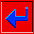## Trend surface analysis

The two general classes of techniques for estimating a regular grid of points on a surface from scattered observations are methods called "global fit" and "local fit." As the name suggests, global-fit procedures calculate a single function describing a surface that covers the entire map area. The function is evaluated to obtain values at the grid nodes. In contrast, local-fit procedures estimate the surface at successive nodes in the grid using only a selection of the nearest data points.

Trend surface analysis is the most widely used global surface-fitting procedure. The mapped data are approximated by a polynomial expansion of the geographic coordinates of the control points, and the coefficients of the polynomial function are found by the method of least squares, insuring that the sum of the squared deviations from the trend surface is a minimum. Each original observation is considered to be the sum of a deterministic polynomial function of the geographic coordinates plus a random error.

The polynomial can be expanded to any desired degree, although there are computational limits because of rounding error. The unknown coefficients are found by solving a set of simultaneous linear equations which include the sums of powers and cross products of the X, Y, and Z values. Once the coefficients have been estimated, the polynomial function can be evaluated at any point within the map area. It is a simple matter to create a grid matrix of values by substituting the coordinates of the grid nodes into the polynomial and calculating an estimate of the surface for each node. Because of the least-squares fitting procedure, no other polynomial equation of the same degree can provide a better approximation of the data.go back